Do you like the article?
Share it with others -

Use new possibilities of MetaTrader 5

# Multi-symbol balance graph in MetaTrader 5

24 May 2018, 13:29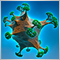0
27 754

### Introduction

In one of the previous articles, we considered visualization of multi-symbol balance graphs. Since then, a lot of MQL libraries have appeared providing an ability to fully implement such a visualization in MetaTrader 5 platform without using third-party programs.

In this article, I will show a sample application with a graphical interface featuring multi-symbol balance graph and deposit drawdowns as a result of the last test. After completing the EA test, a deal history is to be written to a file. These data can then be read and displayed on the graphs.

In addition, the article presents a version of the EA, in which a multi-symbol balance graph is displayed and updated on the graphical interface right during trading, as well as during a test in visualization mode.

### Developing the graphical interface

In the article "Visualizing trading strategy optimization in MetaTrader 5", we have examined in details how to include and use EasyAndFast library and how it can help in developing a graphical interface for your MQL application. Therefore, here we start with the appropriate graphical interface at once.

Let's list the elements to be used in the graphical interface.

• Form for controls.
• Button for updating the graphs with the results of the last test.
• Multi-symbol balance graph.
• Deposit drawdown graph.
• Status bar for displaying additional summary information.

The code listing below provides declarations of methods for creating these elements. Method implementation is performed in a separate include file.

```//+------------------------------------------------------------------+
//| Class for creating the application                               |
//+------------------------------------------------------------------+
class CProgram : public CWndEvents
{
private:
//--- Window
CWindow           m_window1;
//--- Status bar
CStatusBar        m_status_bar;
//--- Graphs
CGraph            m_graph1;
CGraph            m_graph2;
//--- Buttons
CButton           m_update_graph;
//---
public:
//--- Create the graphical interface
bool              CreateGUI(void);
//---
private:
//--- Form
bool              CreateWindow(const string text);
//--- Status bar
bool              CreateStatusBar(const int x_gap,const int y_gap);
//--- Graphs
bool              CreateGraph1(const int x_gap,const int y_gap);
bool              CreateGraph2(const int x_gap,const int y_gap);
//--- Buttons
bool              CreateUpdateGraph(const int x_gap,const int y_gap,const string text);
};
//+------------------------------------------------------------------+
//| Methods for creating control elements                            |
//+------------------------------------------------------------------+
#include "CreateGUI.mqh"
//+------------------------------------------------------------------+```

In this case, the main method of creating the graphical interface will look as follows:

```//+------------------------------------------------------------------+
//| Create the graphical interface                                   |
//+------------------------------------------------------------------+
bool CProgram::CreateGUI(void)
{
//--- Create the form for control elements
if(!CreateWindow("Expert panel"))
return(false);
//--- Create control elements
if(!CreateStatusBar(1,23))
return(false);
if(!CreateGraph1(1,50))
return(false);
if(!CreateGraph2(1,159))
return(false);
if(!CreateUpdateGraph(7,25,"Update data"))
return(false);
//--- Complete GUI creation
CWndEvents::CompletedGUI();
return(true);
}```

As a result, if you compile the EA now and download its graph in the terminal, the current result will look as follows: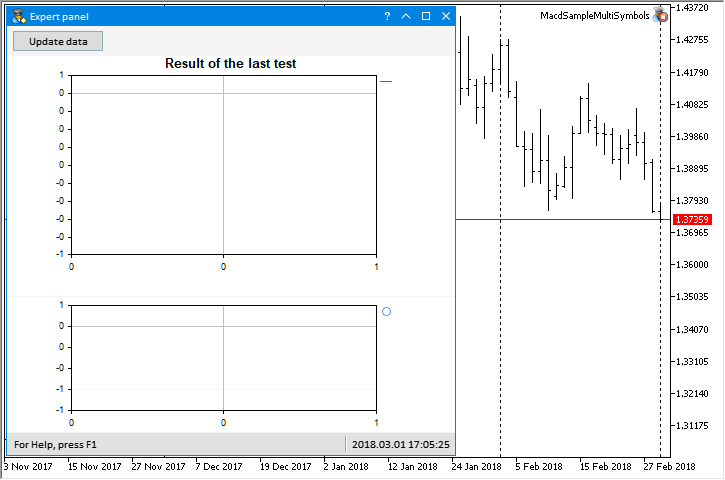Fig. 1. The EA graphical interface

Now, let's consider writing data to a file after the test.

### Multi-symbol EA for tests

To conduct the tests, we will use MACD Sample EA from the standard delivery making it multi-symbol. The multi-symbol structure used in this version is inaccurate. With the same parameters, the result will differ depending on a symbol the test is to be performed on (selected in the tester's settings). Therefore, this EA is intended only for tests and demonstration of the results obtained within the framework of the present topic.

New possibilities for creating multi-symbol EAs will be presented in the nearest MetaTrader 5 updates. Then, it will be possible to think about developing a final and universal version for EAs of this type. If you urgently need a fast and accurate multi-symbol structure, you can try the option proposed on the forum.

Let's add one more string parameter for specifying symbols the test is to be conducted on to the external parameters:

```//--- External parameters
sinput string Symbols           ="EURUSD,USDJPY,GBPUSD,EURCHF"; // Symbols
input  double InpLots           =0.1;                           // Lots
input  int    InpTakeProfit     =167;                           // Take Profit (in pips)
input  int    InpTrailingStop   =97;                            // Trailing Stop Level (in pips)
input  int    InpMACDOpenLevel  =16;                            // MACD open level (in pips)
input  int    InpMACDCloseLevel =19;                            // MACD close level (in pips)
input  int    InpMATrendPeriod  =14;                            // MA trend period```

Symbols are separated by commas. The program class (CProgram) implements methods for reading this parameter as well as for checking symbols and setting in the Market Watch the ones present in the server list. Alternatively, you can specify trading symbols via a preliminarily prepared list in the file as shown in the article "MQL5 Cookbook: Developing a multi-currency Expert Advisor with unlimited number of parameters". Moreover, you can make several lists for a user to choose from. Such an example is provided in the article "MQL5 Cookbook: Reducing the effect of overfitting and handling the lack of quotes". It is possible to come up with many more ways to select symbols and their lists using the graphical interface. I will show a possible option in one of the following articles.

Before testing characters in the common list, we need to save them to an array. Then pass this array (source_array[]) to CProgram::CheckTradeSymbols() method. Here, in the first loop, we pass through symbols specified in the external parameters. In the second loop, we check whether this symbol is on the list on the broker server. If yes, add it to the Market Watch and the array of checked symbols.

If no symbols are detected, only the current symbol the EA is launched at is used.

```class CProgram : public CWndEvents
{
private:
//--- Check trading symbols in a passed array and return the array of available ones
};
//+------------------------------------------------------------------+
//| Check trading symbols in a passed array and                      |
//| and return the array of available ones                           |
//+------------------------------------------------------------------+
{
int symbols_total     =::SymbolsTotal(false);
int size_source_array =::ArraySize(source_array);
//--- Look for specified symbols in a total list
for(int i=0; i<size_source_array; i++)
{
for(int s=0; s<symbols_total; s++)
{
//--- Get the name of the current symbol in the common list
string symbol_name=::SymbolName(s,false);
//--- If there is a match
if(symbol_name==source_array[i])
{
//--- Set a symbol in the market watch
::SymbolSelect(symbol_name,true);
//--- Add to confirmed symbols array
int size_array=::ArraySize(checked_array);
::ArrayResize(checked_array,size_array+1);
checked_array[size_array]=symbol_name;
break;
}
}
}
//--- If no symbols detected, use the current symbol only
if(::ArraySize(checked_array)<1)
{
::ArrayResize(checked_array,1);
checked_array=_Symbol;
}
}```

The CProgram::CheckSymbols() method is used to read an external string parameter symbols are specified in. Here, the string is split into an array using ',' as a separator. The gaps on both sides are cropped in the resulting strings. After that, the array is sent for verification to the CProgram::CheckTradeSymbols() method considered above.

```class CProgram : public CWndEvents
{
private:
//--- Check and include into an array the symbols for trading from the string
int               CheckSymbols(const string symbols_enum);
};
//+-------------------------------------------------------------------------+
//| Check and include into an array the symbols for trading from the string |
//+-------------------------------------------------------------------------+
int CProgram::CheckSymbols(const string symbols_enum)
{
if(symbols_enum!="")
::Print(__FUNCTION__," > input deal symbols: ",symbols_enum);
//--- Get symbols from the string
string symbols[];
ushort u_sep=::StringGetCharacter(",",0);
::StringSplit(symbols_enum,u_sep,symbols);
//--- Crop spaces from both sides
int elements_total=::ArraySize(symbols);
for(int e=0; e<elements_total; e++)
{
::StringTrimLeft(symbols[e]);
::StringTrimRight(symbols[e]);
}
//--- Check the symbols
::ArrayFree(m_symbols);
//--- Get the number of trading symbols
return(::ArraySize(m_symbols));
}```

A file with a trading strategy class is connected to a file with the application class. CStrategy-type dynamic array is created.

```#include "Strategy.mqh"
//+------------------------------------------------------------------+
//| Class for creating the application                               |
//+------------------------------------------------------------------+
class CProgram : public CWndEvents
{
private:
//--- Strategy array
CStrategy         m_strategy[];
};```

Here, we get the array of symbols and their number from the external parameter during the program initialization. Next, set the size for the strategy array by the number of symbols and initialize all strategy instances passing the symbol name to each of them.

```class CProgram : public CWndEvents
{
private:
//--- Total symbols
int               m_symbols_total;
};
//+------------------------------------------------------------------+
//| Initialization                                                   |
//+------------------------------------------------------------------+
bool CProgram::OnInitEvent(void)
{
m_symbols_total=CheckSymbols(Symbols);
//--- TS array size
::ArrayResize(m_strategy,m_symbols_total);
//--- Initialization
for(int i=0; i<m_symbols_total; i++)
{
if(!m_strategy[i].OnInitEvent(m_symbols[i]))
return(false);
}
//--- Initialization successful
return(true);
}```

Next, let's consider writing the last test data to a file.

### Writing data to file

We will save the last test data in the general data folder of the terminals. Thus, the file will be accessible from any MetaTrader 5 platform. Specify the folder and file names in the constructor:

```class CProgram : public CWndEvents
{
private:
//--- Path to file with the last test results
string            m_last_test_report_path;
};
//+------------------------------------------------------------------+
//| Constructor                                                      |
//+------------------------------------------------------------------+
CProgram::CProgram(void) : m_symbols_total(0)
{
//--- Path to file with the last test results
m_last_test_report_path=::MQLInfoString(MQL_PROGRAM_NAME)+"\\LastTest.csv";
}```

Let's consider CProgram::CreateSymbolBalanceReport() method used to write to a file. For working in this method (as well as in another one to be considered later), we will need symbol balance arrays.

```//--- Arrays for balances of all symbols
struct CReportBalance { double m_data[]; };
//+------------------------------------------------------------------+
//| Class for creating the application                               |
//+------------------------------------------------------------------+
class CProgram : public CWndEvents
{
private:
//--- Array of balances of all symbols
CReportBalance    m_symbol_balance[];
//---
private:
//--- Create test report on deals in CSV format
void              CreateSymbolBalanceReport(void);
};
//+------------------------------------------------------------------+
//| Create test report on trades in CSV format                       |
//+------------------------------------------------------------------+
void CProgram::CreateSymbolBalanceReport(void)
{
...
}```

At the beginning of the method, open the file to work in the shared folder of the terminals (FILE_COMMON):

```...
//--- Create a file for writing data in the general terminal folder
int file_handle=::FileOpen(m_last_test_report_path,FILE_CSV|FILE_WRITE|FILE_ANSI|FILE_COMMON);
//--- If the handle is valid (file created/opened)
if(file_handle==INVALID_HANDLE)
{
::Print(__FUNCTION__," > Error creating file: ",::GetLastError());
return;
}
...```

Some auxiliary variables will be needed to form some report parameters. We will write to file the entire history of deals with data provided in the list below:

• Deal time
• Symbol
• Type
• Direction
• Volume
• Price
• Swap
• Result (profit/loss)
• Drawdown
• Balance. This column shows a total balance, while subsequent ones contain balances of symbols used in the test

Here, we form the first line with the data headers:

```...
double max_drawdown    =0.0; // Maximum drawdown
double balance         =0.0; // Balance
string delimeter       =","; // Separator
string string_to_write ="";  // For forming an entry line
...```

If more than one symbol is involved, the header line should be supplemented by their names. After that, headers (first line) should be written to the file

```...
//--- If there is more than one symbol is involved, supplement the header line
int symbols_total=::ArraySize(m_symbols);
if(symbols_total>1)
{
for(int s=0; s<symbols_total; s++)
}
...```

Next, we receive the entire history of deals and their number, setting array sizes:

```...
//--- Get the entire history
::HistorySelect(0,LONG_MAX);
//--- Find out the number of deals
int deals_total=::HistoryDealsTotal();
//--- Set the number of balance arrays by the number of symbols
::ArrayResize(m_symbol_balance,symbols_total);
//--- Set the size of deal arrays for each symbol
for(int s=0; s<symbols_total; s++)
::ArrayResize(m_symbol_balance[s].m_data,deals_total);
...```

In the main loop, pass along the entire history and form strings for writing to the file. When calculating profit, consider swap and commission as well. If there are more than one symbols, we pass through them in the second loop and form a balance for each symbol.

The data are written to the file string by string. The file is closed at the end of the method.
```...
//--- Move along the loop and write data
for(int i=0; i<deals_total; i++)
{
//--- Get deal ticket
if(!m_deal_info.SelectByIndex(i))
continue;
//--- Find out the number of digits in a price
int digits=(int)::SymbolInfoInteger(m_deal_info.Symbol(),SYMBOL_DIGITS);
//--- Calculate total balance
balance+=m_deal_info.Profit()+m_deal_info.Swap()+m_deal_info.Commission();
//--- Form the line for writing by concatenation
::StringConcatenate(string_to_write,
::TimeToString(m_deal_info.Time(),TIME_DATE|TIME_MINUTES),delimeter,
m_deal_info.Symbol(),delimeter,
m_deal_info.TypeDescription(),delimeter,
m_deal_info.EntryDescription(),delimeter,
::DoubleToString(m_deal_info.Volume(),2),delimeter,
::DoubleToString(m_deal_info.Price(),digits),delimeter,
::DoubleToString(m_deal_info.Swap(),2),delimeter,
::DoubleToString(m_deal_info.Profit(),2),delimeter,
MaxDrawdownToString(i,balance,max_drawdown),delimeter,
::DoubleToString(balance,2));
//--- If there are more than one symbol, write their balance values
if(symbols_total>1)
{
//--- Move along all symbols
for(int s=0; s<symbols_total; s++)
{
//--- If symbols match and a deal result is not zero
if(m_deal_info.Symbol()==m_symbols[s] && m_deal_info.Profit()!=0)
//--- Show a trade in the balance with this symbol. Consider swap and commission
m_symbol_balance[s].m_data[i]=m_symbol_balance[s].m_data[i-1]+m_deal_info.Profit()+m_deal_info.Swap()+m_deal_info.Commission();
//--- Otherwise, write the previous value
else
{
//--- In case of a "balance deposit" deal (first deal), the balance is the same for all symbols
if(m_deal_info.DealType()==DEAL_TYPE_BALANCE)
m_symbol_balance[s].m_data[i]=balance;
//--- Otherwise, write the previous value to the current index
else
m_symbol_balance[s].m_data[i]=m_symbol_balance[s].m_data[i-1];
}
//--- Add symbol balance to string
}
}
//--- Write the formed string
::FileWrite(file_handle,string_to_write);
//--- Forcibly set the variable for the next string to zero
string_to_write="";
}
//--- Close the file
::FileClose(file_handle);
...```

When forming strings (see the code below), the CProgram::MaxDrawdownToString() method is used to write to the file for calculating the total balance drawdown. During its first call, the drawdown is equal to zero. The current balance is saved as the local maximum/minimum. During the following method calls, a drawdown is calculated by previous values and the local maximum is updated if the balance exceeds the saved one. Otherwise, the local minimum is updated and zero value (empty string) is returned.

```class CProgram : public CWndEvents
{
private:
//--- Get maximum drawdown from the local maximum
string            MaxDrawdownToString(const int deal_number,const double balance,double &max_drawdown);
};
//+------------------------------------------------------------------+
//| Get maximum drawdown from the local maximum                      |
//+------------------------------------------------------------------+
string CProgram::MaxDrawdownToString(const int deal_number,const double balance,double &max_drawdown)
{
//--- String for displaying in the report
string str="";
//--- For local maximum and drawdown calculation
static double max=0.0;
static double min=0.0;
if(deal_number==0)
{
//--- No drawdown yet
max_drawdown=0.0;
//--- Set the initial point as a local maximum
max=balance;
min=balance;
}
else
{
//--- If the current balance exceeds the saved one
if(balance>max)
{
//--- Calculate drawdown by previous values
max_drawdown=100-((min/max)*100);
//--- Update local maximum
max=balance;
min=balance;
}
else
{
//--- Get zero drawdown and update minimum
max_drawdown=0.0;
min=fmin(min,balance);
}
}
//--- Define string for report
str=(max_drawdown==0)? "" : ::DoubleToString(max_drawdown,2);
return(str);
}```

The file structure allows opening it in Excel (see the screenshot below):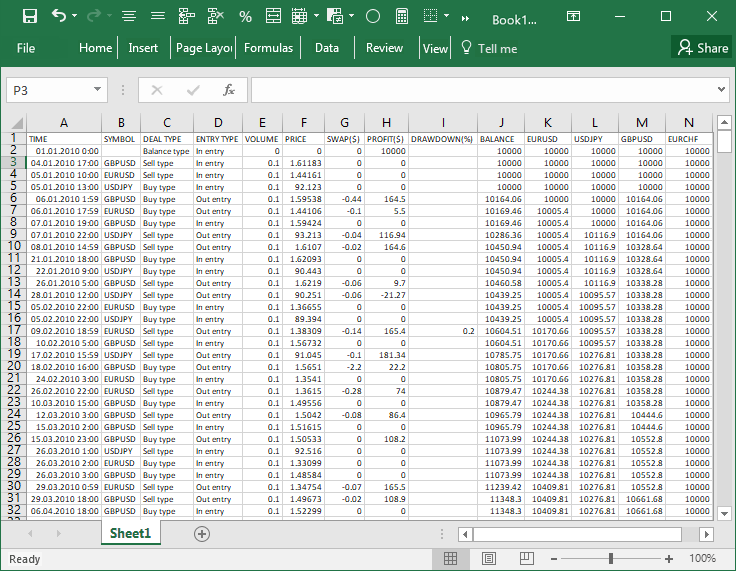Fig. 2. Report file structure in Excel

As a result, the call of the CProgram::CreateSymbolBalanceReport() method for preparing a test report is performed at the end of the test:

```//+------------------------------------------------------------------+
//| Test completion event                                            |
//+------------------------------------------------------------------+
double CProgram::OnTesterEvent(void)
{
//--- Write report only after the test
if(::MQLInfoInteger(MQL_TESTER) && !::MQLInfoInteger(MQL_OPTIMIZATION) &&
!::MQLInfoInteger(MQL_VISUAL_MODE) && !::MQLInfoInteger(MQL_FRAME_MODE))
{
//--- Form report and write to files
CreateSymbolBalanceReport();
}
//---
return(0.0);
}```

Now, let's consider reading the report data.

### Extracting data from file

After all we have implemented above, each EA check in the strategy tetser ends with writing a report to a file. Next, let's consider the methods used to read data from the report. First, we need to read the file and insert its contents to the array to work with it conveniently. To achieve this, we use CProgram::ReadFileToArray() method. Here we open the file the trade history at the end of the EA test was written to. In the loop, read the file till the last string and fill in the array with source data.

```class CProgram : public CWndEvents
{
private:
//--- Array for data from file
string            m_source_data[];
//---
private:
//--- Read file to the passed array
};
//+------------------------------------------------------------------+
//| Read file to the passed array                                    |
//+------------------------------------------------------------------+
{
//--- Open the file
//--- Exit if the file has not opened
if(file_handle==INVALID_HANDLE)
return(false);
//--- Free the array
::ArrayFree(m_source_data);
//--- Read the file to the array
while(!::FileIsEnding(file_handle))
{
int size=::ArraySize(m_source_data);
::ArrayResize(m_source_data,size+1,RESERVE);
}
//--- Close the file
::FileClose(file_handle);
return(true);
}```

We will need the auxiliary CProgram::GetStartIndex() method for defining the BALANCE column index. You can pass to it the dynamic array for the elements of the string split using ',' separator and header string as arguments. In this string, the search for a column name is performed.

```class CProgram : public CWndEvents
{
private:
//--- Initial baLalnce index in the report
};
//+------------------------------------------------------------------+
//| Define the index the data copying starts from                    |
//+------------------------------------------------------------------+
{
//--- Get string elements by the separator
string str_elements[];
ushort u_sep=::StringGetCharacter(",",0);
//--- Search for 'BALANCE' column
int elements_total=::ArraySize(str_elements);
for(int e=elements_total-1; e>=0; e--)
{
string str=str_elements[e];
::StringToUpper(str);
//--- If the column with the necessary header is found
if(str=="BALANCE")
{
m_balance_index=e;
break;
}
}
if(m_balance_index==WRONG_VALUE)
{
::Print(__FUNCTION__," > In the report file, there is no heading \'BALANCE\' ! ");
return(false);
}
//--- Successful
return(true);
}```

Deal numbers are displayed by X axis on both graphs. The range of dates will be displayed in the balance graph footer as an extra info. The CProgram::GetDateRange() method is implemented for defining the start and end dates of the trade history. Two string variables are passed to it by reference to the start and end dates of the trade history.

```class CProgram : public CWndEvents
{
private:
//--- Range of dates
void              GetDateRange(string &from_date,string &to_date);
};
//+------------------------------------------------------------------+
//| Get the start and end dates of the test range                    |
//+------------------------------------------------------------------+
void CProgram::GetDateRange(string &from_date,string &to_date)
{
//--- Exit if there are less than three strings
int strings_total=::ArraySize(m_source_data);
if(strings_total<3)
return;
//--- Get the start and end dates of the report
string str_elements[];
ushort u_sep=::StringGetCharacter(",",0);
//---
::StringSplit(m_source_data,u_sep,str_elements);
from_date=str_elements;
::StringSplit(m_source_data[strings_total-1],u_sep,str_elements);
to_date=str_elements;
}```

The CProgram::GetReportDataToArray() and CProgram::AddDrawDown() methods are used to get balance and drawdown data. The second one called in the first one and its code is very short (see the listing below). The trade index and drawdown value are passed here. The index and the value are inserted into the appropriate arrays, whose values are then displayed on the graph. The drawn value is saved to m_dd_y[], while the index to display this value on is saved to m_dd_x[]. Thus, the graphs based on indices with no values will display nothing (empty values).

```class CProgram : public CWndEvents
{
private:
//--- Drawdown by total balance
double            m_dd_x[];
double            m_dd_y[];
//---
private:
//--- Add the drawdown to the arrays
void              AddDrawDown(const int index,const double drawdown);
};
//+------------------------------------------------------------------+
//| Add the drawdown to the arrays                                   |
//+------------------------------------------------------------------+
void CProgram::AddDrawDown(const int index,const double drawdown)
{
int size=::ArraySize(m_dd_y);
::ArrayResize(m_dd_y,size+1,RESERVE);
::ArrayResize(m_dd_x,size+1,RESERVE);
m_dd_y[size] =drawdown;
m_dd_x[size] =(double)index;
}```

Array sizes and the number of series for the balance graph are first defined in the CProgram::GetReportDataToArray() method. Then initialize the header array. After that, string elements by separator are retrieved in a loop string by string, and the data is placed to the drawdown and balance arrays.

```class CProgram : public CWndEvents
{
private:
//--- Get symbol data from the report
};
//+------------------------------------------------------------------+
//| Get symbol data from the report                                  |
//+------------------------------------------------------------------+
{
string str_elements[];
ushort u_sep=::StringGetCharacter(",",0);
::StringSplit(m_source_data,u_sep,str_elements);
//--- Array sizes
int strings_total  =::ArraySize(m_source_data);
int elements_total =::ArraySize(str_elements);
//--- Free the arrays
::ArrayFree(m_dd_y);
::ArrayFree(m_dd_x);
//--- Get the number of series
int curves_total=elements_total-m_balance_index;
curves_total=(curves_total<3)? 1 : curves_total;
//--- Set the size for arrays by the number of series
::ArrayResize(m_symbol_balance,curves_total);
//--- Set the size of series
for(int i=0; i<curves_total; i++)
::ArrayResize(m_symbol_balance[i].m_data,strings_total,RESERVE);
if(curves_total>2)
{
for(int i=0,e=m_balance_index; e<elements_total; e++,i++)
}
else
//--- Get data
for(int i=1; i<strings_total; i++)
{
::StringSplit(m_source_data[i],u_sep,str_elements);
//--- Gather data to arrays
if(str_elements[m_balance_index-1]!="")
//--- If there are several symbols
if(curves_total>2)
for(int b=0,e=m_balance_index; e<elements_total; e++,b++)
m_symbol_balance[b].m_data[i]=double(str_elements[e]);
else
m_symbol_balance.m_data[i]=double(str_elements[m_balance_index]);
}
//--- The first series value
for(int i=0; i<curves_total; i++)
m_symbol_balance[i].m_data=(strings_total<2)? 0 : m_symbol_balance[i].m_data;
//--- Get the number of series
return(curves_total);
}```

Next, we will consider how to display obtained data on the graphs.

### Displaying data on the graphs

The auxiliary methods considered in the previous section are called at the start of the CProgram::UpdateBalanceGraph() method for updating the balance graph. Then the current series are removed from the graph, since the number of symbols participating in the last test may change. Then add the new balance data series in the loop by the current number of symbols defined in the CProgram::GetReportDataToArray() method and define the minimum and maximum values by Y axis.

Here, we also memorize the size of the series and scale spacing by X axis in the class fields. These values are also needed for formatting the drawdown graph. Indents for graph extreme points equal to 5% are calculated for Y axis. As a result, all these values are applied to the balance graph, while the graph is updated for displaying the recent changes.

```class CProgram : public CWndEvents
{
private:
//--- Total data in the series
double            m_data_total;
//--- Scale spacing on X scale
double            m_default_step;
//---
private:
//--- Update data on the balance graph
void              UpdateBalanceGraph(void);
};
//+------------------------------------------------------------------+
//| Update the balance graph                                         |
//+------------------------------------------------------------------+
void CProgram::UpdateBalanceGraph(void)
{
//--- Get the test range dates
string from_date=NULL,to_date=NULL;
GetDateRange(from_date,to_date);
//--- Define the index the data copying starts from
if(!GetBalanceIndex(m_source_data))
return;
//--- Get symbol data from the report

//--- Update all graph series using new data
CColorGenerator m_generator;
CGraphic *graph=m_graph1.GetGraphicPointer();
//--- Clear the graph
int total=graph.CurvesTotal();
for(int i=total-1; i>=0; i--)
graph.CurveRemoveByIndex(i);
//--- Chart high and low
double y_max=0.0,y_min=m_symbol_balance.m_data;
for(int i=0; i<curves_total; i++)
{
//--- Define high/low by Y axis
y_max=::fmax(y_max,m_symbol_balance[i].m_data[::ArrayMaximum(m_symbol_balance[i].m_data)]);
y_min=::fmin(y_min,m_symbol_balance[i].m_data[::ArrayMinimum(m_symbol_balance[i].m_data)]);
//--- Add series to the graph
}
//--- Number of values and X axis grid step
m_data_total   =::ArraySize(m_symbol_balance.m_data)-1;
m_default_step =(m_data_total<10)? 1 : ::MathFloor(m_data_total/5.0);
//--- Range and indents
double range  =::fabs(y_max-y_min);
double offset =range*0.05;
//--- Color for the first series
graph.CurveGetByIndex(0).Color(::ColorToARGB(clrCornflowerBlue));
//--- Horizontal axis properties
CAxis *x_axis=graph.XAxis();
x_axis.AutoScale(false);
x_axis.Min(0);
x_axis.Max(m_data_total);
x_axis.MaxGrace(0);
x_axis.MinGrace(0);
x_axis.DefaultStep(m_default_step);
x_axis.Name(from_date+" - "+to_date);
//--- Vertical axis properties
CAxis *y_axis=graph.YAxis();
y_axis.AutoScale(false);
y_axis.Min(y_min-offset);
y_axis.Max(y_max+offset);
y_axis.MaxGrace(0);
y_axis.MinGrace(0);
y_axis.DefaultStep(range/10.0);
//--- Update the graph
graph.CurvePlotAll();
graph.Update();
}```

The CProgram::UpdateDrawdownGraph() method is used to update the drawdown graph. Since the data are already calculated in the CProgram::UpdateBalanceGraph() method, here we should simply apply them to the graph and refresh it.

```class CProgram : public CWndEvents
{
private:
//--- Update data on the drawdown graph
void              UpdateDrawdownGraph(void);
};
//+------------------------------------------------------------------+
//| Update the drawdown graph                                        |
//+------------------------------------------------------------------+
void CProgram::UpdateDrawdownGraph(void)
{
//--- Update the drawdown graph
CGraphic *graph=m_graph2.GetGraphicPointer();
CCurve *curve=graph.CurveGetByIndex(0);
curve.Update(m_dd_x,m_dd_y);
curve.PointsFill(false);
curve.PointsSize(6);
curve.PointsType(POINT_CIRCLE);
//--- Horizontal axis properties
CAxis *x_axis=graph.XAxis();
x_axis.AutoScale(false);
x_axis.Min(0);
x_axis.Max(m_data_total);
x_axis.MaxGrace(0);
x_axis.MinGrace(0);
x_axis.DefaultStep(m_default_step);
//--- Update the graph
graph.CalculateMaxMinValues();
graph.CurvePlotAll();
graph.Update();
}```

The CProgram::UpdateBalanceGraph() and CProgram::UpdateDrawdownGraph() methods are called in the CProgram::UpdateGraphs() method. Before calling them, the CProgram::ReadFileToArray() method is called first. It receives data from the file with the EA last test results.

```class CProgram : public CWndEvents
{
private:
//--- Update data on the last test results graphs
void              UpdateGraphs(void);
};
//+------------------------------------------------------------------+
//| Update the graphs                                                |
//+------------------------------------------------------------------+
void CProgram::UpdateGraphs(void)
{
//--- Fill in the array with the data from the file
{
::Print(__FUNCTION__," > Could not open the test results file!");
return;
}
//--- Refresh the balance and drawdown graph
UpdateBalanceGraph();
UpdateDrawdownGraph();
}```

### Displaying the obtained results

To display the results of the last test on the interface graphs, click a single button. The appropriate event is processed in CProgram::OnEvent() method:

```//+------------------------------------------------------------------+
//| Event handler                                                    |
//+------------------------------------------------------------------+
void CProgram::OnEvent(const int id,const long &lparam,const double &dparam,const string &sparam)
{
//--- Button clicking events
if(id==CHARTEVENT_CUSTOM+ON_CLICK_BUTTON)
{
//--- Pressing 'Update data'
if(lparam==m_update_graph.Id())
{
//--- Update the graphs
UpdateGraphs();
return;
}
//---
return;
}
}```

If the EA has already been tested before clicking the button, we will see something like this: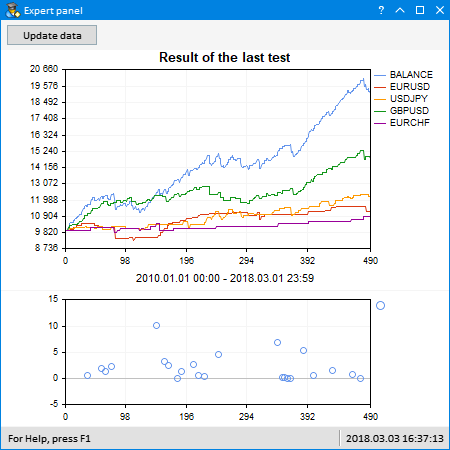Fig. 3. The EA's last test result

Thus, if the EA has been uploaded on the graph, you immediately see the changes on the multi-symbol balance graph, while viewing multiple tests results after parameter optimization.

### Multi-symbol balance graph during trading and tests

Now, let's consider the second EA version, in which the multi-symbol balance graph is displayed and updated during trading.

The graphical interface remains almost the same as in the above version. The only difference is that the refresh button is replaced with a drop-down calendar allowing you to specify a date, from which the trading result is displayed on the graphs.

We will check the history change by the arrival of the event in the OnTrade() method. The CProgram::IsLastDealTicket() method is used to ensure that a new deal has been added to the history. In this method, we will get history from the time saved in the memory after the last call. Then, check the tickets of the last deal and the ticket saved in memory. If the tickets are different, update the saved ticket and the last trade time for the next check, and get 'true' property informing that the history has changed.

```class CProgram : public CWndEvents
{
private:
//--- Time and ticket of the last changed deal
datetime          m_last_deal_time;
ulong             m_last_deal_ticket;
//---
private:
//--- Check the new deal
bool              IsLastDealTicket(void);
};
//+------------------------------------------------------------------+
//| Constructor                                                      |
//+------------------------------------------------------------------+
CProgram::CProgram(void) : m_last_deal_time(NULL),
m_last_deal_ticket(WRONG_VALUE)
{
}
//+------------------------------------------------------------------+
//| Get the last trade event on a specified symbol                   |
//+------------------------------------------------------------------+
bool CProgram::IsLastDealTicket(void)
{
//--- Exit if the story is not received yet
if(!::HistorySelect(m_last_deal_time,LONG_MAX))
return(false);
//--- Get the number of deals in the obtained list
int total_deals=::HistoryDealsTotal();
//--- Go through all deals in the received list from the last to the first one
for(int i=total_deals-1; i>=0; i--)
{
//--- Get a deal ticket
ulong deal_ticket=::HistoryDealGetTicket(i);
//--- If tickets are equal, exit
if(deal_ticket==m_last_deal_ticket)
return(false);
//--- If tickets are not equal, inform of that
else
{
datetime deal_time=(datetime)::HistoryDealGetInteger(deal_ticket,DEAL_TIME);
//--- Save the last deal's time and ticket
m_last_deal_time   =deal_time;
m_last_deal_ticket =deal_ticket;
return(true);
}
}
//--- Tickets of another symbol
return(false);
}
```

Before going through the deal history and filling arrays with data, we should define what symbols are in the history and what their number is to set the size of arrays. To achieve this, we use the CProgram::GetHistorySymbols() method. Before calling it, select history in the desired range. Then, add symbols found in history to the string. To ensure that the symbols are not repeated, check for the specified sub-string. After that, add the symbols detected in history to the array and get the number of symbols.

```class CProgram : public CWndEvents
{
private:
//--- Symbol array from history
string            m_symbols_name[];
//---
private:
//--- Get symbols from account history and return their number
int               GetHistorySymbols(void);
};
//+------------------------------------------------------------------+
//| Get symbols from account history and return their number         |
//+------------------------------------------------------------------+
int CProgram::GetHistorySymbols(void)
{
string check_symbols="";
//--- Go through the loop for the first time and get traded symbols
int deals_total=::HistoryDealsTotal();
for(int i=0; i<deals_total; i++)
{
//--- Get the deal ticket
if(!m_deal_info.SelectByIndex(i))
continue;
//--- If there is a symbol name
if(m_deal_info.Symbol()=="")
continue;
//--- If there is no such a string, add it
if(::StringFind(check_symbols,m_deal_info.Symbol(),0)==-1)
}
//--- Get string elements by separator
ushort u_sep=::StringGetCharacter(",",0);
int symbols_total=::StringSplit(check_symbols,u_sep,m_symbols_name);
//--- Return the number of symbols
return(symbols_total);
}
```

To get a multi-symbol balance, call the CProgram::GetHistorySymbolsBalance() method:

```class CProgram : public CWndEvents
{
private:
//--- Get the total balance and balance per each symbol separately
void              GetHistorySymbolsBalance(void);
};
//+------------------------------------------------------------------+
//| Get the total balance and balance per each symbol separately     |
//+------------------------------------------------------------------+
void CProgram::GetHistorySymbolsBalance(void)
{
...
}```

Here we should get the initial account balance at the very beginning. Get the history for the very first trade. It will be used as the initial balance. It is assumed that it is possible to specify a date in the calendar trading results are displayed from. Therefore, select the history again. Then, use the CProgram::GetHistorySymbols() method to get symbols in the selected history and their number. After that, set the size of the arrays. Define the start and end dates for displaying the history result range.

```...
//--- Initial deposit size
::HistorySelect(0,LONG_MAX);
double balance=(m_deal_info.SelectByIndex(0))? m_deal_info.Profit() : 0;
//--- Get history from the specified date
//--- Get the number of symbols
int symbols_total=GetHistorySymbols();
//--- Free the arrays
::ArrayFree(m_dd_x);
::ArrayFree(m_dd_y);
//--- Set the balance array size by the number of symbols + 1 for the total balance
::ArrayResize(m_symbols_balance,(symbols_total>1)? symbols_total+1 : 1);
//--- Set the size of the deal arrays per each symbol
int deals_total=::HistoryDealsTotal();
for(int s=0; s<=symbols_total; s++)
{
if(symbols_total<2 && s>0)
break;
//---
::ArrayResize(m_symbols_balance[s].m_data,deals_total);
::ArrayInitialize(m_symbols_balance[s].m_data,0);
}
//--- Number of balance curves
int balances_total=::ArraySize(m_symbols_balance);
//--- History start and end
m_end_date   =(m_deal_info.SelectByIndex(deals_total-1))? m_deal_info.Time() : ::TimeCurrent();
...```

Symbol and drawdown balances are calculated in the next loop. Obtained data are placed to the arrays. The methods described in the previous sections are also used here to calculate the drawdown.

```...
//--- Maximum drawdown
double max_drawdown=0.0;
//--- Write balance arrays to the passed array
for(int i=0; i<deals_total; i++)
{
//--- Get the deal array
if(!m_deal_info.SelectByIndex(i))
continue;
//--- Initialize on the first deal
if(i==0 && m_deal_info.DealType()==DEAL_TYPE_BALANCE)
balance=0;
//--- From the specified date
{
//--- Count the total balance
balance+=m_deal_info.Profit()+m_deal_info.Swap()+m_deal_info.Commission();
m_symbols_balance.m_data[i]=balance;
//--- Calculate the drawdown
if(MaxDrawdownToString(i,balance,max_drawdown)!="")
}
//--- Write the symbols' balance values if more than one symbol is used
if(symbols_total<2)
continue;
//--- From the specified date only
continue;
//--- Move through all symbols
for(int s=1; s<balances_total; s++)
{
int prev_i=i-1;
//--- In case of the "Balance deposit" deal (first deal) ...
if(prev_i<0 || m_deal_info.DealType()==DEAL_TYPE_BALANCE)
{
//--- ... the balance is the same for all symbols
m_symbols_balance[s].m_data[i]=balance;
continue;
}
//--- If the symbols are equal and the deal result is not zero
if(m_deal_info.Symbol()==m_symbols_name[s-1] && m_deal_info.Profit()!=0)
{
//--- Reflect the deal in the balance with this symbol. Consider swap and commission.
m_symbols_balance[s].m_data[i]=m_symbols_balance[s].m_data[prev_i]+m_deal_info.Profit()+m_deal_info.Swap()+m_deal_info.Commission();
}
//--- Otherwise, write the previous value
else
m_symbols_balance[s].m_data[i]=m_symbols_balance[s].m_data[prev_i];
}
}
...```

The data are added to graphs and updated using the CProgram::UpdateBalanceGraph() and CProgram::UpdateDrawdownGraph() methods. Their code is almost identical to the one in the first EA version considered in the previous sections, therefore let's move to calling them at once.

First, these methods are called when creating a graphical interface, so that users immediately see a deal result. After that, the graphs are updated when receiving trading events in the OnTrade() method.

```class CProgram : public CWndEvents
{
private:
//--- Initialize graphs
void              UpdateBalanceGraph(const bool update=false);
void              UpdateDrawdownGraph(void);
};
//+------------------------------------------------------------------+
//+------------------------------------------------------------------+
{
//--- Update balance and drawdown graphs
UpdateBalanceGraph();
UpdateDrawdownGraph();
}```

In addition, in the graphical interface, users can specify the date the balance graphs are to be built from. To forcibly refresh the graph without checking the last deal ticket, pass true to the CProgram::UpdateBalanceGraph() method.

Event of changing the date in the calendar (ON_CHANGE_DATE) is processed the following way:

```//+------------------------------------------------------------------+
//| Event handler                                                    |
//+------------------------------------------------------------------+
void CProgram::OnEvent(const int id,const long &lparam,const double &dparam,const string &sparam)
{
//--- Event of selecting date in the calendar
if(id==CHARTEVENT_CUSTOM+ON_CHANGE_DATE)
{
{
UpdateBalanceGraph(true);
UpdateDrawdownGraph();
}
//---
return;
}
}```

Below, you can see how it works in the tester in visualization mode: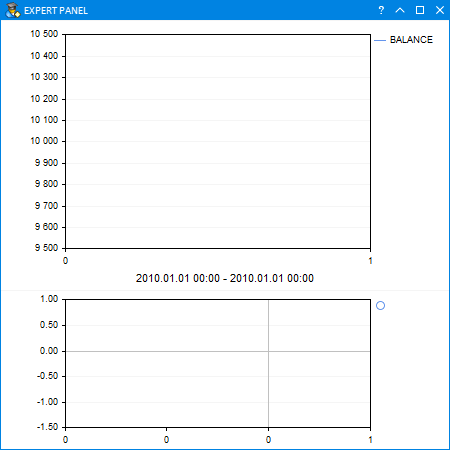Fig. 4. Displaying the tester result in the visualization mode

### Visualizing reports from the Signals service

As another supplement that can be useful for users, we will create an EA enabling visualization of the trading results from reports in the Signals service.

Go to a page of a necessary signal and select "Trading history":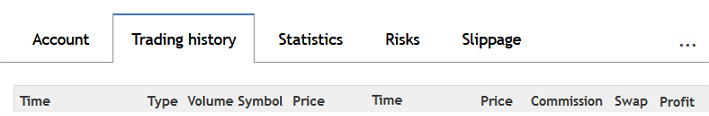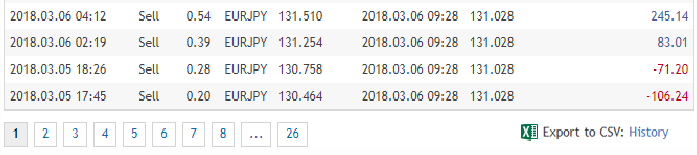Fig. 6. Exporting trade history to the CSV file

These files for the current EA implementation should be placed to \MQL5\Files. Add one external parameters to the EA. It will show the name of the report file, the data of which should be visualized on the graphs.

```//+------------------------------------------------------------------+
//|                                                      Program.mqh |
//|                        Copyright 2018, MetaQuotes Software Corp. |
//|                                              http://www.mql5.com |
//+------------------------------------------------------------------+
//--- External parameters
input string PathToFile=""; // Path to file
...```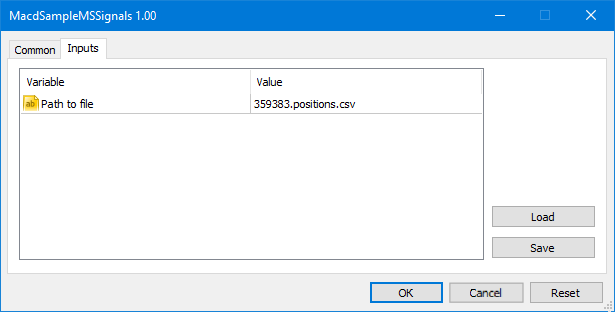Fig. 7. External parameter for specifying the report file

The graphical interface of this EA version contains only two graphs. When launching the EA on the terminal chart, it attempts opening the file specified in the settings. If no such file is found, the program displays a message in the Journal. The set of methods here is about the same as in the versions described above. There are minor differences in some places, but the main principle is the same. Let's consider only the methods where the approach has considerably changed.

So, the file has been read and the strings from it have been placed to the array for source data. Now, you need to distribute this data into a two-dimensional array, as it is done in tables. This is necessary for convenient data sorting by trade open time from the earliest to the latest one. We need a separate array of arrays for this.

```//--- Arrays for data from the file
struct CReportTable
{
string            m_rows[];
};
//+------------------------------------------------------------------+
//| Class for creating the application                               |
//+------------------------------------------------------------------+
class CProgram : public CWndEvents
{
private:
//--- Table for report
CReportTable      m_columns[];
//--- Number of strings and columns
uint              m_rows_total;
uint              m_columns_total;
};
//+------------------------------------------------------------------+
//| Constructor                                                      |
//+------------------------------------------------------------------+
CProgram::CProgram(void) : m_rows_total(0),
m_columns_total(0)
{
...
}```

The following methods are needed for sorting the array of arrays:

```class CProgram : public CWndEvents
{
private:
//--- Fast sorting method
void              QuickSort(uint beg,uint end,uint column);
//--- Check sorting conditions
bool              CheckSortCondition(uint column_index,uint row_index,const string check_value,const bool direction);
//--- Swap values in specified cells
void              Swap(uint r1,uint r2);
};```

All these methods were thoroughly discussed in one of the previous articles

All basic operations are performed in the CProgram::GetData() method. Let us dwell on it in more detail.

```class CProgram : public CWndEvents
{
private:
//--- Get data to arrays
int               GetData(void);
};
//+------------------------------------------------------------------+
//| Get symbol data from the report                                  |
//+------------------------------------------------------------------+
int CProgram::GetData(void)
{
...
}```

First, let's define the number of strings and string elements by ';' separator. Then get symbol names present in the report and their number in a separate array. After that, prepare the arrays and fill them with report data.

```...
string str_elements[];
ushort u_sep=::StringGetCharacter(";",0);
::StringSplit(m_source_data,u_sep,str_elements);
//--- Number of strings and string elements
int strings_total  =::ArraySize(m_source_data);
int elements_total =::ArraySize(str_elements);
//--- Get symbols
if((m_symbols_total=GetHistorySymbols())==WRONG_VALUE)
return;
//--- Free the arrays
::ArrayFree(m_dd_y);
::ArrayFree(m_dd_x);
//--- Data series size
::ArrayResize(m_columns,elements_total);
for(int i=0; i<elements_total; i++)
::ArrayResize(m_columns[i].m_rows,strings_total-1);
//--- Fill in the arrays with data from the file
for(int r=0; r<strings_total-1; r++)
{
::StringSplit(m_source_data[r+1],u_sep,str_elements);
for(int c=0; c<elements_total; c++)
m_columns[c].m_rows[r]=str_elements[c];
}
...```

All is ready for data sorting. Here, we need to set the size of symbol balance arrays before filling them:

```...
//--- Number of series and columns
m_rows_total    =strings_total-1;
m_columns_total =elements_total;
//--- Sort by time in the first column
QuickSort(0,m_rows_total-1,0);
//--- Series size
::ArrayResize(m_symbol_balance,m_symbols_total);
for(int i=0; i<m_symbols_total; i++)
::ArrayResize(m_symbol_balance[i].m_data,m_rows_total);
...```

Then, fill the total balance and drawdowns array. All trades related to replenishing a deposit are skipped.

```...
//--- Balance and maximum drawdown
double balance      =0.0;
double max_drawdown =0.0;
//--- Get total balance data
for(uint i=0; i<m_rows_total; i++)
{
//--- Initial balance
if(i==0)
{
balance+=(double)m_columns[elements_total-1].m_rows[i];
m_symbol_balance.m_data[i]=balance;
}
else
{
//--- Skip replenishments
if(m_columns.m_rows[i]=="Balance")
m_symbol_balance.m_data[i]=m_symbol_balance.m_data[i-1];
else
{
balance+=(double)m_columns[elements_total-1].m_rows[i]+(double)m_columns[elements_total-2].m_rows[i]+(double)m_columns[elements_total-3].m_rows[i];
m_symbol_balance.m_data[i]=balance;
}
}
//--- Calculate the drawdown
if(MaxDrawdownToString(i,balance,max_drawdown)!="")
}
...```

Then fill in balance arrays for each symbol.

```...
//--- Get symbol balance data
for(int s=1; s<m_symbols_total; s++)
{
//--- Initial balance
balance=m_symbol_balance.m_data;
m_symbol_balance[s].m_data=balance;
//---
for(uint r=0; r<m_rows_total; r++)
{
//--- If symbols do not match, then the previous value
if(m_symbols_name[s]!=m_columns[m_symbol_index].m_rows[r])
{
if(r>0)
m_symbol_balance[s].m_data[r]=m_symbol_balance[s].m_data[r-1];
//---
continue;
}
//--- If the deal result is not zero
if((double)m_columns[elements_total-1].m_rows[r]!=0)
{
balance+=(double)m_columns[elements_total-1].m_rows[r]+(double)m_columns[elements_total-2].m_rows[r]+(double)m_columns[elements_total-3].m_rows[r];
m_symbol_balance[s].m_data[r]=balance;
}
//--- Otherwise, write the previous value
else
m_symbol_balance[s].m_data[r]=m_symbol_balance[s].m_data[r-1];
}
}
...```

After that, the data are displayed on the graphs of the graphical interface. Several examples from various signal providers are displayed below: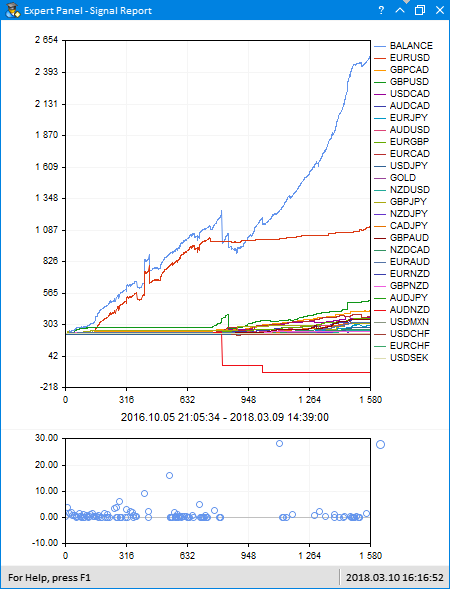Fig. 8. Displaying the results (example 1)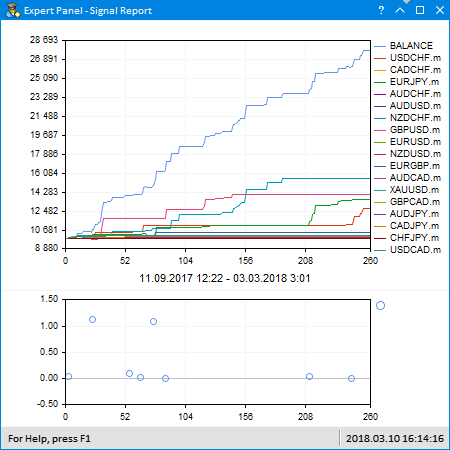Fig. 9. Displaying the results (example 2)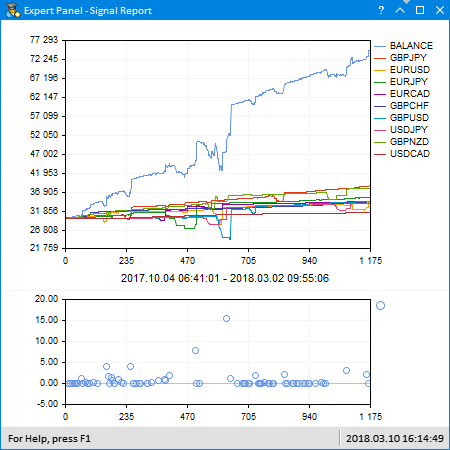Fig. 10. Displaying the results (example 3)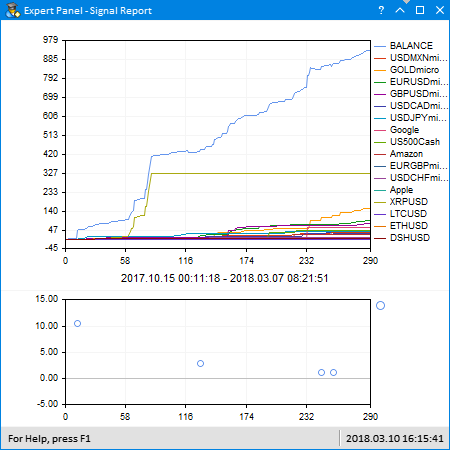Fig. 11. Displaying the results (example 4)

### Conclusion

The article displays the modern version of an MQL application for viewing multi-symbol balance graphs. Previously, you had to use third-party programs to get this result. Now everything can be implemented only with MQL without leaving MetaTrader 5.

Below, you can download the files for testing and detailed study of the code provided in the article. Each program version has the following file structure:

File name Comment
MacdSampleMultiSymbols.mq5 Modified EA from the standard delivery - MACD Sample
Program.mqh File with the program class
CreateGUI.mqh File implementing methods from the program class in Program.mqh file
Strategy.mqh File with the modified MACD Sample strategy class (multi-symbol version)
FormatString.mqh File with auxiliary functions for strings formatting

Translated from Russian by MetaQuotes Software Corp.
Original article: https://www.mql5.com/ru/articles/4430

Attached files |
MQL5.zip (39.77 KB)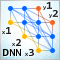Deep Neural Networks (Part V). Bayesian optimization of DNN hyperparameters

The article considers the possibility to apply Bayesian optimization to hyperparameters of deep neural networks, obtained by various training variants. The classification quality of a DNN with the optimal hyperparameters in different training variants is compared. Depth of effectiveness of the DNN optimal hyperparameters has been checked in forward tests. The possible directions for improving the classification quality have been determined.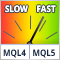Comparing speeds of self-caching indicators

The article compares the classic MQL5 access to indicators with alternative MQL4-style methods. Several varieties of MQL4-style access to indicators are considered: with and without the indicator handles caching. Considering the indicator handles inside the MQL5 core is analyzed as well.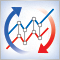Synchronizing several same-symbol charts on different timeframes

When making trading decisions, we often have to analyze charts on several timeframes. At the same time, these charts often contain graphical objects. Applying the same objects to all charts is inconvenient. In this article, I propose to automate cloning of objects to be displayed on charts.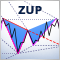ZUP - Universal ZigZag with Pesavento patterns. Search for patterns

The ZUP indicator platform allows searching for multiple known patterns, parameters for which have already been set. These parameters can be edited to suit your requirements. You can also create new patterns using the ZUP graphical interfaces and save their parameters to a file. After that you can quickly check, whether these new patterns can be found on charts.